# RS Aggarwal Class 8 Solutions Chapter 10 Profit and Loss Ex 10C

## RS Aggarwal Class 8 Solutions Chapter 10 Profit and Loss Ex 10C

These Solutions are part of RS Aggarwal Solutions Class 8. Here we have given RS Aggarwal Solutions Class 8 Chapter 10 Profit and Loss Ex 10C.

Other Exercises

Question 1.
Solution:
List price of refrigerator = Rs. 14650
Sales tax = 6%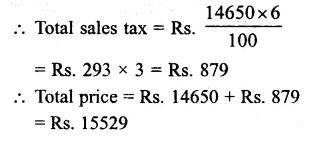Question 2.
Solution:
(i) Lost of tie = Rs. 250
ST = 6%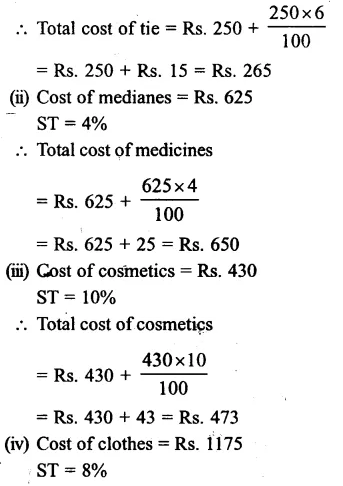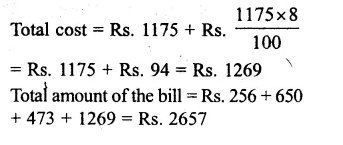Question 3.
Solution:
Price of watch including VAT = Rs. 1980
Rate of VAT = 10%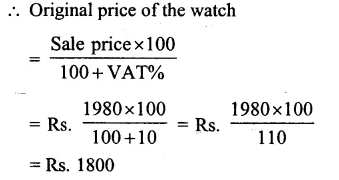Question 4.
Solution:
Price of shirt including VAT = Rs. 133750
Rate of VAT = 7%
∴ Original price of the shirt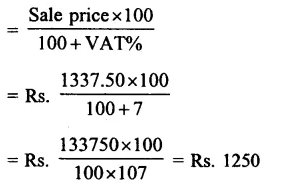Question 5.
Solution:
Sale price of 10 g gold including VAT = Rs. 15756
Rate of VAT = 1%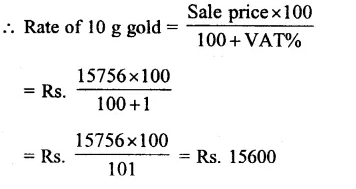Question 6.
Solution:
Sale price of computer including VAT = Rs. 37960
Rate of VAT = 4%
∴ Original price of computer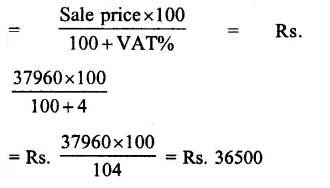Question 7.
Solution:
Sale price of car parts including VAT = Rs. 20776
Rate of VAT = 12%
∴ Original price of car parts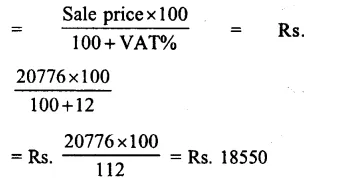Question 8.
Solution:
Sale price of TV set including VAT = Rs. 27000
Rate of VAT = 8%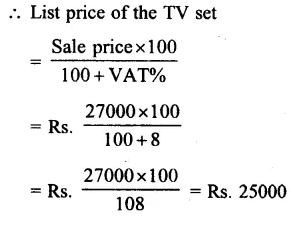Question 9.
Solution:
Sale price of shoes including VAT = Rs. 882
Original price = Rs 840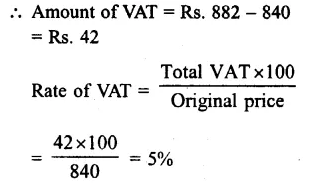Question 10.
Solution:
Sale price of VCR including VAT = Rs. 19980
Original price = Rs. 18500
∴Amount of VAT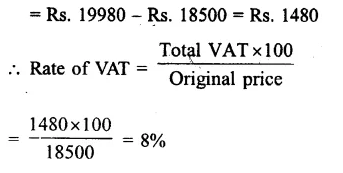Question 11.
Solution:
Sale price of car including VAT = Rs. 382500
Basic price of the car = Rs. 340000
Amount of VAT = Rs. 382500 – 340000
= Rs. 42500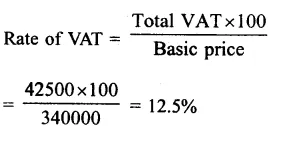Hope given RS Aggarwal Solutions Class 8 Chapter 10 Profit and Loss Ex 10C are helpful to complete your math homework.

If you have any doubts, please comment below. Learn Insta try to provide online math tutoring for you.